Sunday, August 9, 2020
Home > CBSE Class 12 > NCERT Solutions for Class 12 Maths Determinants Exercise 4.6

# NCERT Solutions for Class 12 Maths Determinants Exercise 4.6# NCERT Solutions for Class 12 Maths Determinants

Hi Students, Welcome to Amans Maths Blogs (AMB). In this post, you will get the NCERT Solutions for Class 12 Maths Determinants Exercise 4.6. This NCERT Solutions can be downloaded in PDF file. The downloading link is given at last.

NCERT Solutions for Class 12 Maths are not only the solutions of Maths exercise but it builds your foundation of other important subjects. Getting knowledge of depth concept of CBSE Class 12th Maths like Algebra, Calculus, Trigonometry, Coordinate Geometry help you to understand the concept of Physics and Physical Chemistry.

CBSE Class 12th is an important school class in your life as you take some serious decision about your career. And out of all subjects, Maths is an important and core subjects. So CBSE NCERT Solutions for Class 12th Maths is major role in your exam preparation as it has detailed chapter wise solutions for all exercise.

As we know that all the schools affiliated from CBSE follow the NCERT books for all subjects. You can check the CBSE NCERT Syllabus. Thus, NCERT Solutions helps the students to solve the exercise questions as given in NCERT Books.

## NCERT Solutions for Class 12 Maths Determinants Exercise 4.6

Examine the consistency of the system of equations in Exercises 1 to 6.

NCERT Solutions for Class 12 Maths Determinants Exercise 4.6: Ques No 1.

x + 2y = 2

2x + 3y = 3

NCERT Solutions:

The system of equation can be written in the form of AX = B, whereHence, the system of equation is consistent.

NCERT Solutions for Class 12 Maths Determinants Exercise 4.6: Ques No 2.

2x – y = 5

x + y = 4

NCERT Solutions:

The system of equation can be written in the form of AX = B, where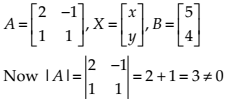Hence, the system of equation is consistent.

NCERT Solutions for Class 12 Maths Determinants Exercise 4.6: Ques No 3.

x + 3y = 5

2x + 6y = 8

NCERT Solutions:

The system of equation can be written in the form of AX = B, where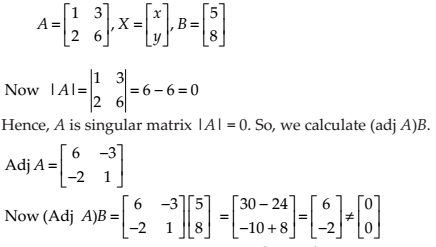Hence, the system of equation is inconsistent with no solution.

NCERT Solutions for Class 12 Maths Determinants Exercise 4.6: Ques No 4.

x + y + z = 1

2x + 3y + 2z = 2

ax + ay + 2az = 4

NCERT Solutions:

The system of equation can be written in the form of AX = B, where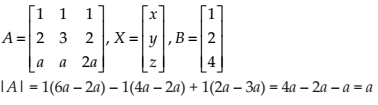Now, there are two cases:

Case I: If |A| = a ≠ 0, then the system of equation is consistent with unique solution.

Case II: If |A| = a = 0, then we need to calculate adjA.Hence, the system of equation is inconsistent with no solutions.

NCERT Solutions for Class 12 Maths Determinants Exercise 4.6: Ques No 5.

3x–y – 2z = 2

2y – z = –1

3x – 5y = 3

NCERT Solutions:

The system of equation can be written in the form of AX = B, where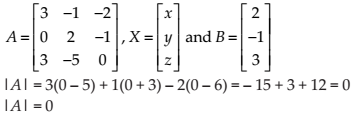Now, A is a singular matrix. So, we need to calculate adjA.Hence, the system of equation is inconsistent with no solutions.

NCERT Solutions for Class 12 Maths Determinants Exercise 4.6: Ques No 6.

5x – y + 4z = 5

2x + 3y + 5z = 2

5x – 2y + 6z = –1

NCERT Solutions:

The system of equation can be written in the form of AX = B, whereHence, the system of equation is consistent with unique solutions.

Solve system of linear equations, using matrix method, in Exercises 7 to 14.

NCERT Solutions for Class 12 Maths Determinants Exercise 4.6: Ques No 7.

5x + 2y = 4

7x + 3y = 5

NCERT Solutions:

The system of equation can be written in the form of AX = B, whereThus, A is non-singular matrix, hence its inverse matrix exist.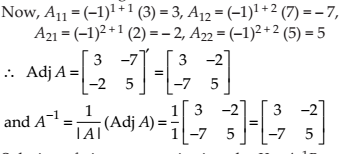Now, the solution of the given equation of system is X = A-1B.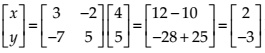Hence, x = 2 and y = -3.

NCERT Solutions for Class 12 Maths Determinants Exercise 4.6: Ques No 8.

2x – y = –2

3x + 4y = 3

NCERT Solutions:

The system of equation can be written in the form of AX = B, where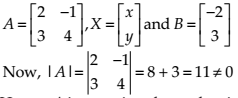Thus, A is non-singular matrix, hence its inverse matrix exist.Now, the solution of the given equation of system is X = A-1B.Hence, x = -5/11 and y = 12/11.

NCERT Solutions for Class 12 Maths Determinants Exercise 4.6: Ques No 9.

4x – 3y = 3

3x – 5y = 7

NCERT Solutions:

The system of equation can be written in the form of AX = B, where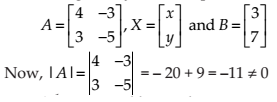Thus, A is non-singular matrix, hence its inverse matrix exist.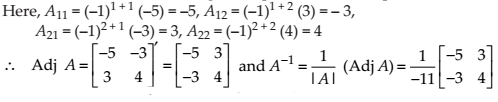Now, the solution of the given equation of system is X = A-1B.Hence, x = -6/11 and y = -19/11.

NCERT Solutions for Class 12 Maths Determinants Exercise 4.6: Ques No 10.

5x + 2y = 3

3x + 2y = 5

NCERT Solutions:

The system of equation can be written in the form of AX = B, where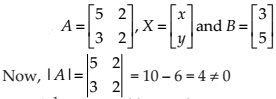Thus, A is non-singular matrix, hence its inverse matrix exist.Now, the solution of the given equation of system is X = A-1B.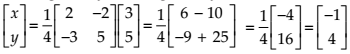Hence, x = -1 and y = 4.

NCERT Solutions for Class 12 Maths Determinants Exercise 4.6: Ques No 11.

2x + y + z = 1

x – 2y – z = 3/2

3y – 5z = 9

NCERT Solutions:

The system of equation can be written in the form of AX = B, where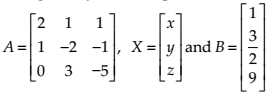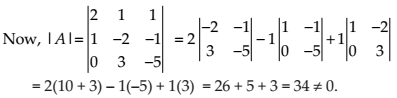Thus, A is non-singular matrix, hence its inverse matrix exist.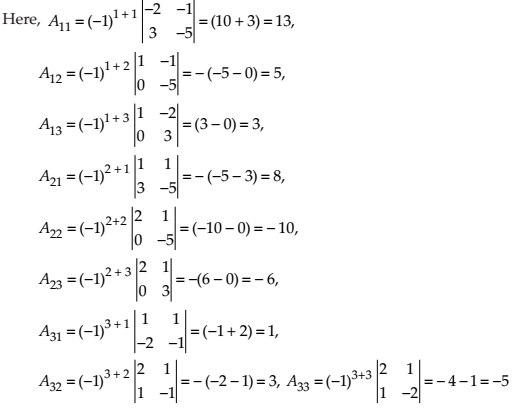Now, the solution of the given equation of system is X = A-1B.Hence, x = 1, y = 1/2 and z = -3/2.

NCERT Solutions for Class 12 Maths Determinants Exercise 4.6: Ques No 12.

x – y + z = 4

2x + y – 3z = 0

x + y + z = 2

NCERT Solutions:

The system of equation can be written in the form of AX = B, where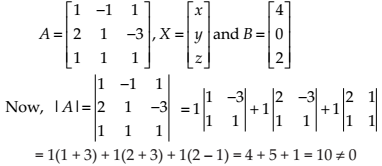Thus, A is non-singular matrix, hence its inverse matrix exist.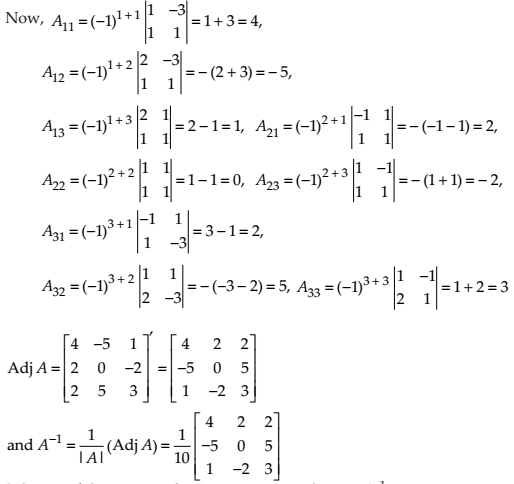Now, the solution of the given equation of system is X = A-1B.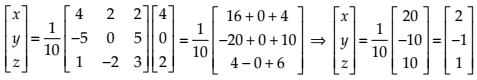Hence, x = 2, y = -1 and z = 1.

NCERT Solutions for Class 12 Maths Determinants Exercise 4.6: Ques No 13.

2x + 3y +3 z = 5

x – 2y + z = – 4

3x – y – 2z = 3

NCERT Solutions:

The system of equation can be written in the form of AX = B, where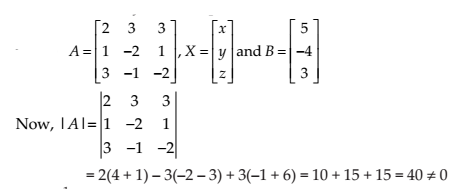Thus, A is non-singular matrix, hence its inverse matrix exist.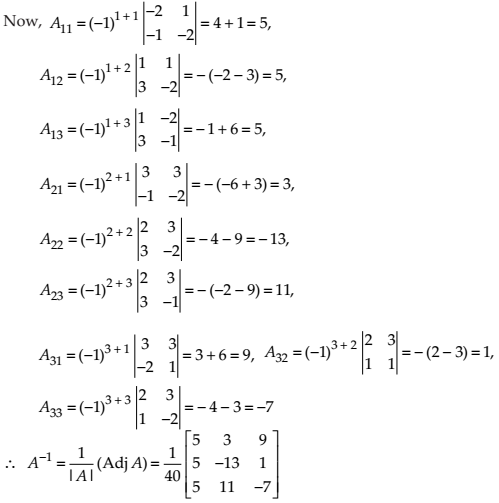Now, the solution of the given equation of system is X = A-1B.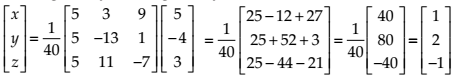Hence, x = 1, y = 2 and z = 1.

NCERT Solutions for Class 12 Maths Determinants Exercise 4.6: Ques No 14.

x – y + 2z = 7

3x + 4y – 5z = – 5

2x – y + 3z = 12

NCERT Solutions:

The system of equation can be written in the form of AX = B, where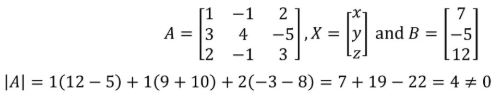Thus, A is non-singular matrix, hence its inverse matrix exist.Now, the solution of the given equation of system is X = A-1B.Hence, x = 1, y = 1 and z = 3.

NCERT Solutions for Class 12 Maths Determinants Exercise 4.6: Ques No 15.

If A =, find A–1. Using A–1 solve the system of equations

2x – 3y + 5z = 11

3x + 2y – 4z = – 5

x + y – 2z = – 3

NCERT Solutions:

The system of equation can be written in the form of AX = B, whereThus, A is non-singular matrix, hence its inverse matrix exist.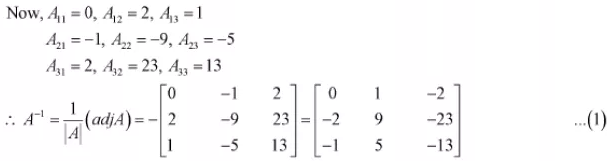Now, the solution of the given equation of system is X = A-1B.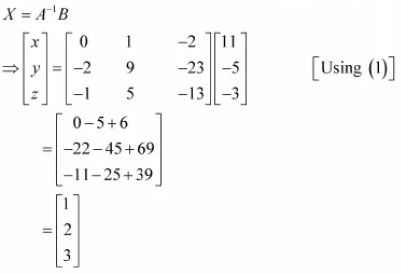Hence, x = 1, y = 2 and z = 3.

NCERT Solutions for Class 12 Maths Determinants Exercise 4.6: Ques No 16.

The cost of 4 kg onion, 3 kg wheat and 2 kg rice is Rs 60. The cost of 2 kg onion, 4 kg wheat and 6 kg rice is Rs 90. The cost of 6 kg onion 2 kg wheat and 3 kg rice is Rs 70. Find cost of each item per kg by matrix method.

NCERT Solutions:

Let the cost of 1 kg onion = Rs. x, the cost of 1 kg wheat = Rs. y, the cost of 1 kg rice = Rs. z.

Then,

4x + 3y + 2z = 60,

2x + 4y + 6z = 90,

6x + 2y + 3z = 70

The system of equation can be written in the form of AX = B, where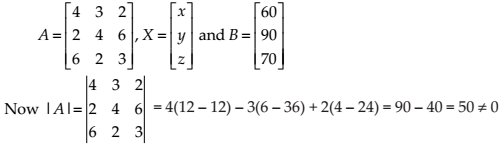Thus, A is non-singular matrix, hence its inverse matrix exist.Now, the solution of the given equation of system is X = A-1B.Hence, x = 5, y = 8 and z = 8.

Thus, the cost of 1 kg onion = Rs. 5, the cost of 1 kg wheat = Rs. 8, the cost of 1 kg rice = Rs. 8.

error: Content is protected !!